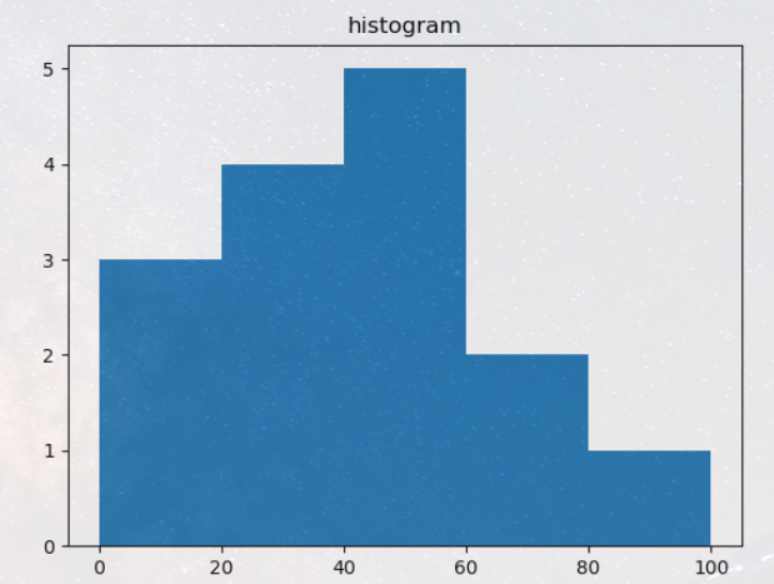# Numpy基础教程

2021/8/20 21:00:28 浏览：### 文章目录

• Numpy
• 简单例子
• 数组创建
• 打印数组
• 基本操作
• 通函数
• 索引、切片和迭代
• 形状操纵
• 改变数组的形状
• 将不同数组堆叠在一起
• 将一个数组拆分成几个较小的数组（分割）
• 纵向分割
• 横向分割
• 错误的分割
• 不等量的分割
• 拷贝和视图
• 完全不复制
• 视图或浅拷贝
• 深拷贝
• 功能和方法概述
• Less 基础
• 花式索引和索引技巧
• 使用索引数组进行索引
• 使用布尔数组进行索引
• ix_()函数
• 简单数组操作
• 技巧和提示
• “自动”整形
• 矢量堆叠
• 直方图
• 数据类型
• 数组标量
• 溢出错误
• 扩展精度
• 创建数组
• 简介
• 将Python array_like对象转换为Numpy数组
• Numpy原生数组的创建
• 从磁盘读取数组
• 标准二进制格式
• 常见ASCII格式
• 自定义二进制格式
• 使用特殊库
• NumPy&Matplotlib
• 直线图
• 绘制正弦波
• subplot()
• bar()
• numpy.histogram()
• plt()

# Numpy

``````%matplotlib inline
``````

NumPy的主要对象是同构多维数组。它是一个元素表（通常是数字），所有类型都相同，由非负整数元组索引。在NumPy维度中称为轴。

``````import numpy as np
import torch
import sys
``````

NumPy的数组类被调用ndarray。它也被别名所知 array。请注意，numpy.array这与标准Python库类不同array.array，后者只处理一维数组并提供较少的功能。ndarray对象更重要的属性是：

• ndarray.ndim - 数组的轴（维度）的个数。在Python世界中，维度的数量被称为rank。
• ndarray.shape - 数组的维度。这是一个整数的元组，表示每个维度中数组的大小。对于有 n 行和 m 列的矩阵，shape 将是 (n,m)。因此，shape 元组的长度就是rank或维度的个数 ndim。
• ndarray.size - 数组元素的总数。这等于 shape 的元素的乘积。
• ndarray.dtype - 一个描述数组中元素类型的对象。可以使用标准的Python类型创建或指定dtype。另外NumPy提供它自己的类型。例如numpy.int32、numpy.int16和numpy.float64。
• ndarray.itemsize - 数组中每个元素的字节大小。例如，元素为 float64 类型的数组的 itemsize 为8（=64/8），而 complex32 类型的数组的 itemsize 为4（=32/8）。它等于 ndarray.dtype.itemsize 。
• ndarray.data - 该缓冲区包含数组的实际元素。通常，我们不需要使用此属性，因为我们将使用索引访问数组中的元素

# 简单例子

``````b = torch.arange(15).reshape(3, 5)
b,b.type
``````
``````(tensor([[ 0,  1,  2,  3,  4],
[ 5,  6,  7,  8,  9],
[10, 11, 12, 13, 14]]),
<function Tensor.type>)
``````
``````array1 = np.array([[1, 2, 3], [4, 5, 6]], dtype=np.float32)  # array.dtype - 一个描述数组中元素类型的对象
a = np.arange(15).reshape(3, 5)
print(a)
print('数组的轴（维度）的个数:', a.ndim)  # array.ndim - 数组的轴（维度）的个数
print('数组的维度:', a.shape)  # array.shape - 数组的维度
print('数组元素的总数:', a.size)  # array.size - 数组元素的总数
print('数组中每个元素的字节大小:', a.itemsize)  # array.itemsize - 数组中每个元素的字节大小
print('数组中元素类型的对象:', a.dtype.name)  # a.dtype.name
print('numpy于pytorch区分', type(a), type(b))
``````
``````[[ 0  1  2  3  4]
[ 5  6  7  8  9]
[10 11 12 13 14]]

numpy于pytorch区分 <class 'numpy.ndarray'> <class 'torch.Tensor'>
``````
• 得到的数组的类型是从Python列表中元素的类型推导出来的。

• 一个常见的错误，就是调用array的时候传入多个数字参数，而不是提供单个数字的列表类型作为参数。

a = np.array(1,2,3,4) # WRONG
a = np.array([1,2,3,4]) # RIGHT

• array 还可以将序列的序列转换成二维数组，将序列的序列的序列转换成三维数组，等等。

# 数组创建

``````a1 = np.array([[1, 2], [3, 4], [5, 6]], dtype=np.float64)
a1,a1.dtype,a1.dtype.name
``````
``````(array([[1., 2.],
[3., 4.],
[5., 6.]]),
dtype('float64'),
'float64')
``````

``````np.zeros((3, 4)),np.ones((3, 4), dtype=np.int64)'''''',np.empty((2, 3),dtype=np.float64) #未初始化，输出可能会发生变化
``````
``````(array([[0., 0., 0., 0.],
[0., 0., 0., 0.],
[0., 0., 0., 0.]]),
array([[1, 1, 1, 1],
[1, 1, 1, 1],
[1, 1, 1, 1]], dtype=int64),
array([[0.3, 0.6, 0.9],
[1.2, 1.5, 1.8]]))
``````

``````# 从第一个参数开始到第二个参数这个区间内，左闭右开，每个数据差第三个参数的大小,步长（step）
np.arange(10,30,5),np.arange(0,2,0.3) #它接受浮点参数
``````
``````(array([10, 15, 20, 25]), array([0. , 0.3, 0.6, 0.9, 1.2, 1.5, 1.8]))
``````

``````from numpy import pi
np.linspace(0,2,9) #从 0 到 2 的 9 个数字
``````
``````array([0.  , 0.25, 0.5 , 0.75, 1.  , 1.25, 1.5 , 1.75, 2.  ])
``````
``````x=np.linspace(0,2*pi,100) #对在很多点评估功能很有用
f = np.sin(x)
``````

[外链图片转存失败,源站可能有防盗链机制,建议将图片保存下来直接上传(img-3ioBb6Jp-1629463429281)(images/1.png)]

# 打印数组

• 最后一个轴从左到右打印，
• 倒数第二个从上到下打印，
• 其余部分也从上到下打印，每个切片用空行分隔。

``````a,a1
``````
``````array([[ 0,  1,  2,  3,  4],
[ 5,  6,  7,  8,  9],
[10, 11, 12, 13, 14]])
``````
``````c = np.arange(12).reshape(4,3,1)
c
``````
``````array([[[ 0],
[ 1],
[ 2]],

[[ 3],
[ 4],
[ 5]],

[[ 6],
[ 7],
[ 8]],

[[ 9],
,
]])
``````

``````np.arange(10000),np.arange(10000).reshape(100,100)
``````
``````(array([   0,    1,    2, ..., 9997, 9998, 9999]),
array([[   0,    1,    2, ...,   97,   98,   99],
[ 100,  101,  102, ...,  197,  198,  199],
[ 200,  201,  202, ...,  297,  298,  299],
...,
[9700, 9701, 9702, ..., 9797, 9798, 9799],
[9800, 9801, 9802, ..., 9897, 9898, 9899],
[9900, 9901, 9902, ..., 9997, 9998, 9999]]))
``````

``````np.set_printoptions(threshold=sys.maxsize) #sys 模块应该被导入
``````

# 基本操作

``````A = np.array([1,2,3])
B = np.arange(3)
A,B
``````
``````(array([1, 2, 3]), array([0, 1, 2]))
``````
``````C = A-B
C
``````
``````array([1, 1, 1])
``````
``````B*2
``````
``````array([0, 2, 4])
``````
``````B**2 #矩阵平方
``````
``````array([0, 1, 4], dtype=int32)
``````
``````10*np.sin(A) #10乘sin(A)中的所有元素
``````
``````array([8.41470985, 9.09297427, 1.41120008])
``````
``````A<35
``````
``````array([ True,  True,  True]
``````

``````A*B #按元素进行运算
``````
``````array([0, 2, 6])
``````
``````A@B #矩阵乘积
``````
``````8
``````
``````A.dot(B)
``````
``````8
``````
``````x = np.array([[1,2],[3,4]])y = np.arange(4).reshape(2,2)x,y
``````
``````(array([[1, 2],        [3, 4]]), array([[0, 1],        [2, 3]]))
``````
``````x*y #按元素进行运算
``````
``````array([[ 0,  2],       [ 6, 12]])
``````
``````x@y #矩阵乘积
``````
``````array([[ 4,  7],       [ 8, 15]])
``````
``````x.dot(y) #矩阵乘积
``````
``````array([[ 4,  7],       [ 8, 15]])
``````

``````a = np.ones((2,3),dtype=np.int64)a
``````
``````array([[1, 1, 1],       [1, 1, 1]], dtype=int64)
``````
``````b = np.random.random((2,3))b
``````
``````array([[0.26364226, 0.41650548, 0.75173233],       [0.80333677, 0.51686147, 0.68512001]])
``````
``````a *= 3a
``````
``````array([[3, 3, 3],       [3, 3, 3]], dtype=int64)
``````
``````b += ab
``````
``````array([[3.26364226, 3.41650548, 3.75173233],       [3.80333677, 3.51686147, 3.68512001]])
``````
``````a +=  b #b 不会自动转换为整数类型
``````
``````---------------------------------------------------------------------------UFuncTypeError                            Traceback (most recent call last)<ipython-input-97-294cacd62d6f> in <module>----> 1 a += b

UFuncTypeError: Cannot cast ufunc 'add' output from dtype('float64') to dtype('int64') with casting rule 'same_kind'
``````

``````a = np.ones(3,dtype=np.int32)b = np.linspace(0,pi,3)a,b,a.dtype,b.dtype
``````
``````(array([1, 1, 1]), array([0.        , 1.57079633, 3.14159265]), dtype('int32'), dtype('float64'))
``````
``````c=a+bc
``````
``````array([1.        , 2.57079633, 4.14159265])
``````
``````c.dtype
``````
``````dtype('float64')
``````
``````d = np.exp(c*1j)d,d.dtype #新的复数类型（complex、complex64和complex128）简化了特定的数学操作。
``````
``````(array([ 0.54030231+0.84147098j, -0.84147098+0.54030231j,        -0.54030231-0.84147098j]), dtype('complex128'))
``````

``````a = np.random.random((2,3))a
``````
``````array([[0.10327828, 0.17525785, 0.74043585],       [0.38620688, 0.61422516, 0.35102992]])
``````
``````a.sum()
``````
``````2.3704339434850485
``````
``````a.min(),a.max()
``````
``````(0.10327827636483311, 0.7404358495396605)
``````

``````b = np.arange(12).reshape(3,4)b
``````
``````array([[ 0,  1,  2,  3],       [ 4,  5,  6,  7],       [ 8,  9, 10, 11]])
``````
``````b.sum(axis=0),b.sum(axis=1) #0为列，1为行
``````
``````(array([12, 15, 18, 21]), array([ 6, 22, 38]))
``````
``````b.min(axis=0),b.min(axis=1),b.max(axis=0),b.max(axis=1)
``````
``````(array([0, 1, 2, 3]), array([0, 4, 8]), array([ 8,  9, 10, 11]), array([ 3,  7, 11]))
``````

Numpy中也具有`cumsum()`函数，其用法如下：

``````b.cumsum(),b.cumsum(axis=0),b.cumsum(axis=1) #将上一个（行，列）向下加合
``````
``````(array([ 0,  1,  3,  6, 10, 15, 21, 28, 36, 45, 55, 66], dtype=int32), array([[ 0,  1,  2,  3],        [ 4,  6,  8, 10],        [12, 15, 18, 21]], dtype=int32), array([[ 0,  1,  3,  6],        [ 4,  9, 15, 22],        [ 8, 17, 27, 38]], dtype=int32))
``````

`cumsum()`函数中：生成的每一项矩阵元素均是从原矩阵首项累加到对应项的元素之和。比如元素9，在`cumsum()`生成的矩阵中序号为3，即原矩阵中2，3，4三个元素的和。

``````print(np.diff(b))
``````

# 通函数

NumPy提供熟悉的数学函数，例如sin，cos和exp。在NumPy中，这些被称为“通函数”（ufunc）。在NumPy中，这些函数在数组上按元素进行运算，产生一个数组作为输出。

``````b = np.arange(3)
b
``````
``````array([0, 1, 2])
``````
``````np.exp(b)   #指数
``````
``````array([1.        , 2.71828183, 7.3890561 ])
``````
``````np.sqrt(b)  #平方
``````
``````array([0.        , 1.        , 1.41421356])
``````
``````c = np.array([1,2,3])
``````
``````(array([1, 3, 5]), array([1, 3, 5]))
``````

# 索引、切片和迭代

``````a = np.arange(10)**3
a,a,a[2:5] #一般都是左闭右开
``````
``````(array([  0,   1,   8,  27,  64, 125, 216, 343, 512, 729], dtype=int32),
8,
array([ 8, 27, 64], dtype=int32))
``````
``````a[:6:2] = -1000 #等同于a[0:6:2] = -1000，从开始到位置 6，将每第二个元素设置为 -1000
a
``````
``````array([-1000,     1, -1000,    27, -1000,   125,   216,   343,   512,
729], dtype=int32)
``````
``````a[ : :-1]   #颠倒数组，倒置
``````
``````array([  729,   512,   343,   216,   125, -1000,    27, -1000,     1,
-1000], dtype=int32)
``````
``````for i in a:
print(i**(1/3.))
``````
``````nan
1.0
nan
3.0
nan
5.0
5.999999999999999
6.999999999999999
7.999999999999999
8.999999999999998

<ipython-input-155-a01b03b90f1f>:2: RuntimeWarning: invalid value encountered in power
print(i**(1/3.))
``````

``````def f(x,y):
return 10*x+y

b = np.fromfunction(f,(5,4),dtype=int)
b,b[2,3],b[0:5,1],b[:,1],b[1:3,:]
``````
``````(array([[ 0,  1,  2,  3],
[10, 11, 12, 13],
[20, 21, 22, 23],
[30, 31, 32, 33],
[40, 41, 42, 43]]),
23,
array([ 1, 11, 21, 31, 41]),
array([ 1, 11, 21, 31, 41]),
array([[10, 11, 12, 13],
[20, 21, 22, 23]]))
``````

``````b[-1]
``````
``````array([40, 41, 42, 43])
``````

b[i] 方括号中的表达式 i 被视为后面紧跟着 : 的多个实例，用于表示剩余轴。NumPy也允许你使用三个点写为 b[i,…]。

• x[1,2,…] 相当于 x[1,2,:,:,:]，
• x[…,3] 等效于 x[:,:,:,:,3]
• x[4,…,5,:] 等效于 x[4,:,:,5,:]。
``````c = np.array([[[0,1,2],[10,12,13]],[[100,101,102],[110,112,113]]])
c.shape
``````
``````(2, 2, 3)
``````
``````c[1,...] #与 c[1,:,:] 或 c 相同
``````
``````array([[100, 101, 102],
[110, 112, 113]])
``````
``````c[...,2] #与 c[:,:,2] 相同
``````
``````array([[  2,  13],       [102, 113]])
``````

``````for row in b:    print(row)
``````
``````[0 1 2 3][10 11 12 13][20 21 22 23][30 31 32 33][40 41 42 43]
``````

``````for element in b.flat:    print(element)
``````
``````012310111213202122233031323340414243
``````

# 形状操纵

## 改变数组的形状

``````a = np.floor(10*np.random.random((3,4)))aa.shape
``````
``````(3, 4)
``````
``````a.ravel() #返回数组，扁平化
``````
``````array([0., 9., 7., 1., 1., 4., 3., 7., 5., 9., 5., 2.])
``````
``````a.reshape(6,2) #返回具有修改形状的数组
``````
``````array([[0., 9.],       [7., 1.],       [1., 4.],       [3., 7.],       [5., 9.],       [5., 2.]])
``````
``````a.T #返回数组，转置
``````
``````array([[0., 1., 5.],       [9., 4., 9.],       [7., 3., 5.],       [1., 7., 2.]])
``````
``````a.T.shape ,a.shape
``````
``````((4, 3), (3, 4))
``````

``````a
``````
``````array([[0., 9., 7., 1.],       [1., 4., 3., 7.],       [5., 9., 5., 2.]])
``````
``````a.resize((2,6))a
``````
``````array([[0., 9., 7., 1., 1., 4.],       [3., 7., 5., 9., 5., 2.]])
``````

``````a.reshape(3,-1)
``````
``````array([[0., 9., 7., 1.],       [1., 4., 3., 7.],       [5., 9., 5., 2.]])
``````

# 将不同数组堆叠在一起

``````a = np.floor(10*np.random.random((2,2)))a
``````
``````array([[9., 1.],       [8., 0.]])
``````
``````b = np.floor(10*np.random.random((2,2)))b
``````
``````array([[4., 9.],       [3., 1.]])
``````
``````np.vstack((a,b)),np.hstack((a,b)) #vstack上下堆叠，hstack左右堆叠
``````
``````(array([[9., 1.],        [8., 0.],        [4., 9.],        [3., 1.]]), array([[9., 1., 4., 9.],        [8., 0., 3., 1.]]))
``````

np.newaxis的作用就是选取部分的数据增加一个维度

``````array=random.rand(4,4)
``````
``````array([[0.45284467, 0.27883581, 0.72870975, 0.03455946],       [0.74005136, 0.52413785, 0.78433733, 0.80114353],       [0.16559874, 0.56112999, 0.18464461, 0.38968731],       [0.05684794, 0.50929997, 0.45789637, 0.63199181]])
``````
``````array_add_aix=array[:,np.newaxis]
``````
``````array([[[0.45284467, 0.27883581, 0.72870975, 0.03455946]],        [[0.74005136, 0.52413785, 0.78433733, 0.80114353]],        [[0.16559874, 0.56112999, 0.18464461, 0.38968731]],        [[0.05684794, 0.50929997, 0.45789637, 0.63199181]]])
``````

``````>>> array_add_axis=array[0:2,np.newaxis]>>> array_add_axisarray([[[0.45284467, 0.27883581, 0.72870975, 0.03455946]],       [[0.74005136, 0.52413785, 0.78433733, 0.80114353]]])
``````

``````from numpy import newaxis np.column_stack((a,b))
``````
``````array([[9., 1., 4., 9.],       [8., 0., 3., 1.]])
``````
``````a = np.array([4.,2.])b = np.array([3.,8.])np.column_stack((a,b))     # 返回一个二维数组
``````
``````array([[4., 3.],       [2., 8.]])
``````
``````np.hstack((a,b)),np.vstack((a,b))
``````
``````(array([4., 2., 3., 8.]), array([[4., 2.],        [3., 8.]]))
``````
``````a[:,newaxis],b[:,newaxis]
``````
``````(array([[4.],        [2.]]), array([[3.],        [8.]]))
``````
``````np.column_stack((a[:,newaxis],b[:,newaxis])),np.hstack((a[:,newaxis],b[:,newaxis]))
``````
``````(array([[4., 3.],        [2., 8.]]), array([[4., 3.],        [2., 8.]]))
``````

``````C = np.concatenate((A,B,B,A),axis=0)C
``````
``````array([,       ,       ,       ,       ,       ,       ,       ,       ,       ,       ,       ])
``````
``````D = np.concatenate((A,B,B,A),axis=1)D
``````
``````array([[1, 2, 2, 1],       [1, 2, 2, 1],       [1, 2, 2, 1]])
``````

`axis`参数很好的控制了矩阵的纵向或是横向打印，相比较`vstack``hstack`函数显得更加方便。

``````np.r_[1:4,0,4]
``````
``````array([1, 2, 3, 0, 4])
``````

# 将一个数组拆分成几个较小的数组（分割）

## 纵向分割

``````A = np.arange(12).reshape((3, 4))np.split(A, 2, axis=1)
``````
``````[array([[0, 1],        [4, 5],        [8, 9]]), array([[ 2,  3],        [ 6,  7],        [10, 11]])]
``````

## 横向分割

``````np.split(A, 3, axis=0)
``````
``````[array([[0, 1, 2, 3]]), array([[4, 5, 6, 7]]), array([[ 8,  9, 10, 11]])]
``````

## 错误的分割

``````np.split(A, 3, axis=1)
``````
``````# ValueError: array split does not result in an equal division
``````

## 不等量的分割

``````np.array_split(A, 3, axis=1)
``````
``````[array([[0, 1],        [4, 5],        [8, 9]]), array([[ 2],        [ 6],        ]), array([[ 3],        [ 7],        ])]
``````

``````a = np.floor(10*np.random.random((2,12)))a
``````
``````array([[4., 6., 4., 6., 6., 3., 5., 0., 0., 6., 3., 7.],       [8., 9., 0., 5., 8., 1., 7., 7., 7., 9., 2., 9.]])
``````
``````np.hsplit(a,3)   # 将 a 拆分为 3部分
``````
``````[array([[4., 6., 4., 6.],        [8., 9., 0., 5.]]), array([[6., 3., 5., 0.],        [8., 1., 7., 7.]]), array([[0., 6., 3., 7.],        [7., 9., 2., 9.]])]
``````
``````np.hsplit(a,(3,4)) #在第三和第四列之后拆分 a
``````
``````[array([[4., 6., 4.],        [8., 9., 0.]]), array([[6.],        [5.]]), array([[6., 3., 5., 0., 0., 6., 3., 7.],        [8., 1., 7., 7., 7., 9., 2., 9.]])]
``````

vsplit沿垂直轴分割，并array_split允许指定要分割的轴。

# 拷贝和视图

## 完全不复制

``````a = np.arange(12)a
``````
``````array([ 0,  1,  2,  3,  4,  5,  6,  7,  8,  9, 10, 11])
``````
``````b = a #没有创建新对象b
``````
``````array([ 0,  1,  2,  3,  4,  5,  6,  7,  8,  9, 10, 11])
``````
``````b is a,a.shape
``````
``````(True, (12,))
``````
``````b.shape = 3,4 #改变a的形状a.shape
``````
``````(3, 4)
``````

Python将可变对象作为引用传递，因此函数调用不会复制。

``````def f(x):    print(id(x))    id(a),f(a) #id 是对象的唯一标识符
``````
``````1833900046576(1833900046576, None)
``````

## 视图或浅拷贝

``````c = a.view() #c是a拥有的数据的视图c,c is a ,c.base is a
``````
``````(array([[ 0,  1,  2,  3],
[ 4,  5,  6,  7],
[ 8,  9, 10, 11]]),
False,
True)
``````
``````c.flags.owndata
``````
``````False
``````
``````c.shape = 2,6 #a 的形状不会改变
a.shape
``````
``````(3, 4)
``````
``````c[0,4] = 1234
a
``````
``````array([[   0,    1,    2,    3],
[1234,    5,    6,    7],
[   8,    9,   10,   11]])
``````

``````s = a[ : , 1:3]  #为清晰起见添加了空格； 也可以写成“s = a[:,1:3]”
s
``````
``````array([[ 1,  2],
[ 5,  6],
[ 9, 10]])
``````
``````s[:]=10  #s[:] 是 s 的一个视图。 注意 s=10 和 s[:]=10 的区别
s,a
``````
``````(array([[10, 10],
[10, 10],
[10, 10]]),
array([[   0,   10,   10,    3],
[1234,   10,   10,    7],
[   8,   10,   10,   11]]))
``````

## 深拷贝

``````d = a.copy() #创建一个带有新数据的新数组对象
d is a,d.base is a #d 不与 a 共享任何东西
``````
``````(False, False)
``````
``````d[0,0] =111
a
``````
``````array([[   0,   10,   10,    3],
[1234,   10,   10,    7],
[   8,   10,   10,   11]])
``````

``````a = np.arange(int(1e8))
b = a[:100].copy()
del a #可以释放``a`` 的内存。
``````

# 功能和方法概述

• 数组的创建（Array Creation） - arange, array, copy, empty, empty_like, eye, fromfile, fromfunction, identity, linspace, logspace, mgrid, ogrid, ones, ones_like, zeros, zeros_like
• 转换和变换（Conversions） - ndarray.astype, atleast_1d, atleast_2d, atleast_3d, mat
• 操纵术（Manipulations） - array_split, column_stack, concatenate, diagonal, dsplit, dstack, hsplit, hstack, ndarray.item, newaxis, ravel, repeat, reshape, resize, squeeze, swapaxes, take, transpose, vsplit, vstack
• 询问（Questions） - all, any, nonzero, where,
• 顺序（Ordering） - argmax, argmin, argsort, max, min, ptp, searchsorted, sort
• 操作（Operations） - choose, compress, cumprod, cumsum, inner, ndarray.fill, imag, prod, put, putmask, real, sum
• 基本统计（Basic Statistics） - cov, mean, std, var
• 基本线性代数（Basic Linear Algebra） - cross, dot, outer, linalg.svd, vdot

`nonzero()`函数：

1

``````A = np.arange(2,14).reshape((3,4))
# array([[ 2, 3, 4, 5]
#        [ 6, 7, 8, 9]
#        [10,11,12,13]])
print(np.nonzero(A))
``````
``````(array([0,0,0,0,1,1,1,1,2,2,2,2]),array([0,1,2,3,0,1,2,3,0,1,2,3]))
``````

# Less 基础

## 花式索引和索引技巧

NumPy提供比常规Python序列更多的索引功能。除了通过整数和切片进行索引之外，正如我们之前看到的，数组可以由整数数组和布尔数组索引。

### 使用索引数组进行索引

``````a = np.arange(12)**2  #前 12 个数的平方i = np.array([1,1,3,8,5])  #索引数组a,a[i] #a 在位置 i 的元素
``````
``````(array([  0,   1,   4,   9,  16,  25,  36,  49,  64,  81, 100, 121],       dtype=int32), array([ 1,  1,  9, 64, 25], dtype=int32))
``````
``````j = np.array( [ [ 3, 4], [ 9, 7 ] ] ) #一个二维索引数组a[j]  #与 j 的形状相同
``````
``````array([[ 9, 16],       [81, 49]], dtype=int32)
``````

``````palette = np.array([[0,0,0],                    [255,0,0],                    [0,255,0],                    [0,0,255],                    [255,255,255]])palette
``````
``````array([[  0,   0,   0],       [255,   0,   0],       [  0, 255,   0],       [  0,   0, 255],       [255, 255, 255]])
``````
``````image = np.array([[0,1,2,0],[0,3,4,0]])  #每个值对应于调色板中的一种颜色image
``````
``````array([[0, 1, 2, 0],       [0, 3, 4, 0]])
``````
``````palette[image] #(2,4,3) 彩色图像
``````
``````array([[[  0,   0,   0],        [255,   0,   0],        [  0, 255,   0],        [  0,   0,   0]],       [[  0,   0,   0],        [  0,   0, 255],        [255, 255, 255],        [  0,   0,   0]]])
``````

``````a = np.arange(12).reshape(3,4)a
``````
``````array([[ 0,  1,  2,  3],       [ 4,  5,  6,  7],       [ 8,  9, 10, 11]])
``````
``````i = np.array([[0,1],[1,2]])j = np.array([[2,1],[3,3]])a[i,j] #每个索引各出一个值
``````
``````array([[ 2,  5],       [ 7, 11]])
``````
``````a[i,2],a[:,j]
``````
``````(array([[ 2,  6],        [ 6, 10]]), array([[[ 2,  1],         [ 3,  3]],         [[ 6,  5],         [ 7,  7]],         [[10,  9],         [11, 11]]]))
``````

``````l = [i,j]l
``````
``````[array([[0, 1],        [1, 2]]), array([[2, 1],        [3, 3]])]
``````

``````a[tuple(l)] #防止出错
``````
``````array([[ 2,  5],       [ 7, 11]])
``````

`argmin()``argmax()` 两个函数分别对应着求矩阵中最小元素和最大元素的索引。使用数组索引的另一个常见用法是搜索与时间相关的系列的最大值：

``````time = np.linspace(20, 145, 5) data = np.sin(np.arange(20)).reshape(5,4) time,data
``````
``````(array([ 20.  ,  51.25,  82.5 , 113.75, 145.  ]), array([[ 0.        ,  0.84147098,  0.90929743,  0.14112001],        [-0.7568025 , -0.95892427, -0.2794155 ,  0.6569866 ],        [ 0.98935825,  0.41211849, -0.54402111, -0.99999021],        [-0.53657292,  0.42016704,  0.99060736,  0.65028784],        [-0.28790332, -0.96139749, -0.75098725,  0.14987721]]))
``````
``````ind = data.argmax(axis=0) #每个系列的最大值索引ind
``````
``````array([2, 0, 3, 1], dtype=int64)
``````
``````time_max = time[ind] #???data_max = data[ind, range(data.shape)] # => data[ind,0], data[ind,1]...time_max,data_max
``````
``````(array([ 82.5 ,  20.  , 113.75,  51.25]), array([0.98935825, 0.84147098, 0.99060736, 0.6569866 ]))
``````
``````np.all(data_max == data.max(axis=0))
``````
``````True
``````

``````a = np.arange(5)a
``````
``````array([0, 1, 2, 3, 4])
``````
``````a[[1,3,4]]=0a
``````
``````array([0, 0, 2, 0, 0])
``````

``````a = np.arange(5)a[[0,0,2]]=[1,2,3]a
``````
``````array([2, 1, 3, 3, 4])
``````

``````a = np.arange(5)a[[0,0,2]]+=1a
``````
``````array([1, 1, 3, 3, 4])
``````

``````a = np.arange(3, 15).reshape((3, 4))
``````
``````array([[ 3,  4,  5,  6],       [ 7,  8,  9, 10],       [11, 12, 13, 14]])
``````
``````print(np.mean(a))        # 8.5
``````
``````print(np.average(a))     # 8.5
``````

``````print(a.mean())          # 8.5
``````

``````print(a.median())       # 7.5
``````

``````A = np.arange(3,15).reshape((3,4))print(A.flatten())
``````
``````# array([3, 4, 5, 6, 7, 8, 9, 10, 11, 12, 13, 14])
``````
``````for item in A.flat:	print(item)
``````
``````34567891011121314
``````

# 使用布尔数组进行索引

``````a = np.arange(12).reshape(3,4)b = a > 4b #b 是具有 a 形状的布尔值
``````
``````array([[False, False, False, False],       [False,  True,  True,  True],       [ True,  True,  True,  True]])
``````
``````a[b]  #具有选定元素的一维数组
``````
``````array([ 5,  6,  7,  8,  9, 10, 11])
``````
``````a[b] = 0   #'a' 中大于 4 的所有元素都变为 0a
``````
``````array([[0, 1, 2, 3],
[4, 0, 0, 0],
[0, 0, 0, 0]])
``````

``````import matplotlib.pyplot as plt
def mandelbrot(h,w,maxit=20):
'''返回大小为 (h,w) 的 Mandelbrot 分形图像'''
y,x = np.ogrid[ -1.4:1.4:h*1j, -2:0.8:w*1j ]
c = x+y*1j
z = c
divtime = maxit + np.zeros(z.shape, dtype=int)

for i in range(maxit):
z = z**2 + c
diverge = z*np.conj(z) > 2**2 #谁在发散
div_now = diverge & (divtime==maxit) #现在谁在发散
divtime[div_now] = i #注意什么时候
z[diverge] = 2  #避免发散太多

return divtime
plt.imshow(mandelbrot(400,400))
plt.show()
``````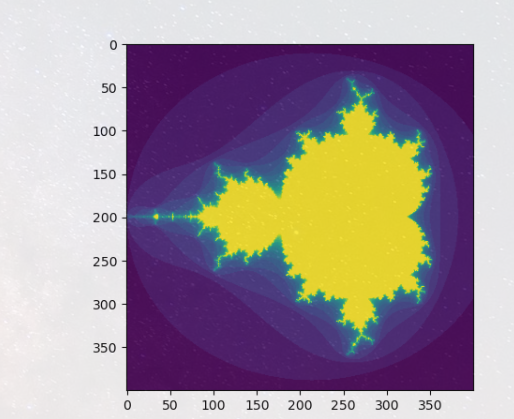``````a = np.arange(12).reshape(3,4)
b1 = np.array([False,True,True])
b2 = np.array([True,False,True,False])
a[b1,:] #选择行
``````
``````array([[ 4,  5,  6,  7],
[ 8,  9, 10, 11]])
``````
``````a[b1] #选择行
``````
``````array([[ 4,  5,  6,  7],       [ 8,  9, 10, 11]])
``````
``````a[:,b2] #选择列
``````
``````array([[ 0,  2],       [ 4,  6],       [ 8, 10]])
``````
``````a[b1,b2]
``````
``````array([ 4, 10])
``````

# ix_()函数

ix_函数可用于组合不同的向量，以便获得每个n-uplet的结果。例如，如果要计算从每个向量a，b和c中取得的所有三元组的所有a + b * c：

``````a = np.array([2,3,4,5])
b = np.array([8,5,4])
c = np.array([5,4,6,8,3])
ax,bx,cx = np.ix_(a,b,c)
ax,bx,cx
``````
``````(array([[],

[],

[],

[]]),
array([[,
,
]]),
array([[[5, 4, 6, 8, 3]]]))
``````
``````ax.shape, bx.shape, cx.shape
``````
``````((4, 1, 1), (1, 3, 1), (1, 1, 5))
``````
``````result = ax+bx*cx
result,a,b,c
``````
``````(array([[[42, 34, 50, 66, 26],
[27, 22, 32, 42, 17],
[22, 18, 26, 34, 14]],

[[43, 35, 51, 67, 27],
[28, 23, 33, 43, 18],
[23, 19, 27, 35, 15]],

[[44, 36, 52, 68, 28],
[29, 24, 34, 44, 19],
[24, 20, 28, 36, 16]],

[[45, 37, 53, 69, 29],
[30, 25, 35, 45, 20],
[25, 21, 29, 37, 17]]]),
array([2, 3, 4, 5]),
array([8, 5, 4]),
array([5, 4, 6, 8, 3]))
``````
``````result[3,2,4]
``````
``````17
``````
``````a+b*c
``````
``````17
``````
``````def ufunc_reduce(ufct, *vectors):
vs = np.ix_(*vectors)
r = ufct.identity
for v in vs:
r = ufct(r,v)
return r
``````
``````ufunc_reduce(np.add,a,b,c) #交叉索引必须是一维的
``````
``````array([[[15, 14, 16, 18, 13],
[12, 11, 13, 15, 10],
[11, 10, 12, 14,  9]],

[[16, 15, 17, 19, 14],
[13, 12, 14, 16, 11],
[12, 11, 13, 15, 10]],

[[17, 16, 18, 20, 15],
[14, 13, 15, 17, 12],
[13, 12, 14, 16, 11]],

[[18, 17, 19, 21, 16],
[15, 14, 16, 18, 13],
[14, 13, 15, 17, 12]]])
``````

## 简单数组操作

``````a = np.array([[1.0, 2.0], [3.0, 4.0]])a
``````
``````array([[1., 2.],       [3., 4.]])
``````
``````a.transpose(),a.T #转置 pirnt(np.transpose(a))
``````
``````(array([[1., 3.],        [2., 4.]]), array([[1., 3.],        [2., 4.]]))
``````
``````np.linalg.inv(a) #矩阵求逆 ，np.linalg.det()：矩阵求行列式（标量）
``````
``````array([[-2. ,  1. ],       [ 1.5, -0.5]])
``````

（1）N:int型，表示的是输出的行数

（2）M：int型，可选项，输出的列数，如果没有就默认为N

（3）k：int型，可选项，对角线的下标，默认为0表示的是主对角线，负数表示的是低对角，正数表示的是高对角。

（4）dtype：数据的类型，可选项，返回的数据的数据类型

（5）order：{‘C’，‘F’}，可选项，也就是输出的数组的形式是按照C语言的行优先’C’，还是按照

``````u = np.eye(2)  u
``````
``````array([[1., 0.],       [0., 1.]])
``````
``````j = np.array([[0.0, -1.0], [1.0, 0.0]])j @ j
``````
``````array([[-1.,  0.],       [ 0., -1.]])
``````
``````np.trace(u) #????
``````
``````2.0
``````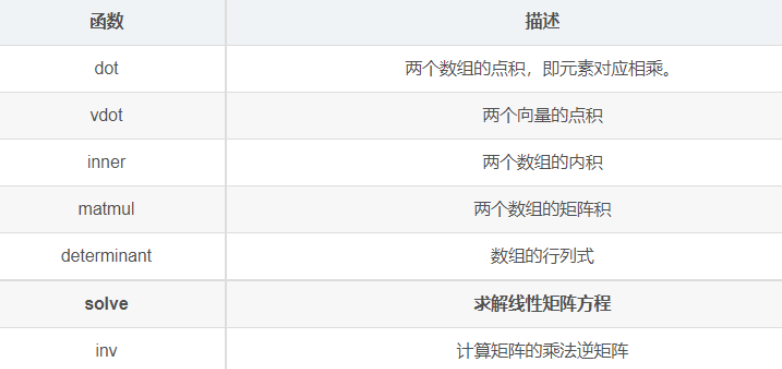``````y = np.array([[5.], [7.]])np.linalg.solve(a, y)
``````
``````array([[-3.],       [ 4.]])
``````
``````np.linalg.eig(j) #求矩阵的特征值与特征向量(np.linalg.eig)
``````
``````(array([0.+1.j, 0.-1.j]), array([[0.70710678+0.j        , 0.70710678-0.j        ],        [0.        -0.70710678j, 0.        +0.70710678j]]))
``````

# 技巧和提示

## “自动”整形

``````a = np.arange(30)a.shape = 2,-1,3a.shape,a
``````
``````((2, 5, 3), array([[[ 0,  1,  2],         [ 3,  4,  5],         [ 6,  7,  8],         [ 9, 10, 11],         [12, 13, 14]],         [[15, 16, 17],         [18, 19, 20],         [21, 22, 23],         [24, 25, 26],         [27, 28, 29]]]))
``````

``````A = np.arange(2,14).reshape((3,4))

# array([[ 2, 3, 4, 5]
#        [ 6, 7, 8, 9]
#        [10,11,12,13]])
``````
``````A = np.arange(14,2, -1).reshape((3,4))
``````
``````array([[14, 13, 12, 11],
[10,  9,  8,  7],
[ 6,  5,  4,  3]])
``````
``````print(np.sort(A))
``````
``````array([[11,12,13,14]
[ 7, 8, 9,10]
[ 3, 4, 5, 6]])
``````

``````print(A)
``````
``````# array([[14,13,12,11]
#        [10, 9, 8, 7]
#        [ 6, 5, 4, 3]])
``````
``````print(np.clip(A,5,9))
``````
``````# array([[ 9, 9, 9, 9]
#        [ 9, 9, 8, 7]
#        [ 6, 5, 5, 5]])
``````

## 矢量堆叠

`vertical stack`本身属于一种上下合并，即对括号中的两个整体进行对应操作。例如：

``````x = np.arange(0,10,2)
y = np.arange(5)
m = np.vstack([x,y])
xy = np.hstack([x,y])
x,y,m,xy
``````
``````(array([0, 2, 4, 6, 8]),
array([0, 1, 2, 3, 4]),
array([[0, 2, 4, 6, 8],
[0, 1, 2, 3, 4]]),
array([0, 2, 4, 6, 8, 0, 1, 2, 3, 4]))
``````

## 直方图

histogram应用于数组的NumPy 函数返回一对向量：数组的直方图和bin的向量。注意： matplotlib还有一个构建直方图的功能（hist在Matlab中称为），与NumPy中的直方图不同。主要区别在于pylab.hist自动绘制直方图，而 numpy.histogram只生成数据。

``````import matplotlib.pyplot as plt'''构建一个包含 10000 个正态偏差的向量，方差为 0.5^2，均值为 2'''mu, sigma = 2, 0.5v = np.random.normal(mu,sigma,10000)'''绘制具有 50 个 bin 的归一化直方图'''plt.hist(v, bins=50, density=1)  plt.show()
``````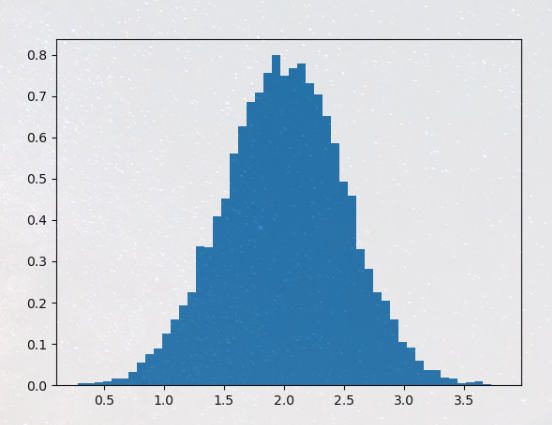``````'''用 numpy 计算直方图，然后绘制它'''(n, bins) = np.histogram(v, bins=50, density=True)plt.plot(.5*(bins[1:]+bins[:-1]), n)plt.show()
``````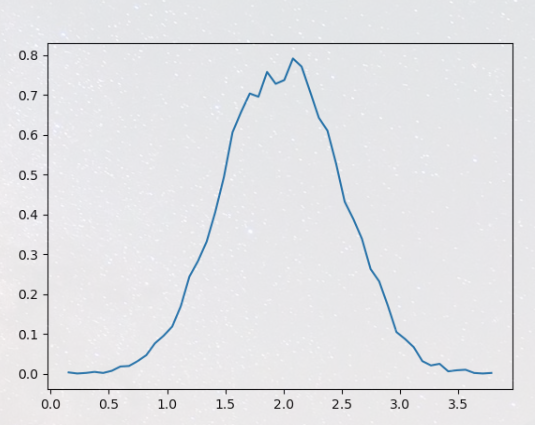# 数据类型

``````x = np.float32(1.0)y = np.int_([1,2,4])z = np.arange(3, dtype=np.uint8)x,y,z
``````
``````(1.0, array([1, 2, 4]), array([0, 1, 2], dtype=uint8))
``````
``````np.array([1, 2, 3], dtype='f')
``````
``````array([1., 2., 3.], dtype=float32)
``````
``````z.astype(float)
``````
``````array([0., 1., 2.])
``````
``````np.int8(z),z.dtype
``````
``````(array([0, 1, 2], dtype=int8), dtype('uint8'))
``````

NumPy的人都知道int是指np.int_，bool意味着np.bool_，这float是np.float_和complex是np.complex_。其他数据类型没有Python等价物。

dtype对象还包含有关类型的信息，例如其位宽和字节顺序。数据类型也可以间接用于查询类型的属性，例如它是否为整数：

``````d = np.dtype(int)d,np.issubdtype(d, np.integer),np.issubdtype(d, np.floating)
``````
``````(dtype('int32'), True, False)
``````

## 数组标量

NumPy通常将数组元素作为数组标量返回（带有关联dtype的标量）。数组标量与Python标量不同，但在大多数情况下它们可以互换使用（主要的例外是早于v2.x的Python版本，其中整数数组标量不能作为列表和元组的索引）。有一些例外，例如当代码需要标量的非常特定的属性或者它特定地检查值是否是Python标量时。通常，存在的问题很容易被显式转换数组标量到Python标量，采用相应的Python类型的功能（例如，固定的int，float，complex，str，unicode）。

## 溢出错误

``````>>> np.power(100, 8, dtype=np.int64)
10000000000000000
>>> np.power(100, 8, dtype=np.int32)
1874919424
``````

NumPy和Python整数类型的行为在整数溢出方面存在显着差异，并且可能会使用户期望NumPy整数的行为类似于Python int。与 NumPy 不同，Python 的大小int 是灵活的。这意味着Python整数可以扩展以容纳任何整数并且不会溢出。

NumPy分别提供numpy.iinfo并numpy.finfo验证NumPy整数和浮点值的最小值或最大值：

``````>>> np.iinfo(np.int) # Bounds of the default integer on this system.
iinfo(min=-9223372036854775808, max=9223372036854775807, dtype=int64)
>>> np.iinfo(np.int32) # Bounds of a 32-bit integer
iinfo(min=-2147483648, max=2147483647, dtype=int32)
>>> np.iinfo(np.int64) # Bounds of a 64-bit integer
iinfo(min=-9223372036854775808, max=9223372036854775807, dtype=int64)
``````

``````>>> np.power(100, 100, dtype=np.int64) # Incorrect even with 64-bit int
0
>>> np.power(100, 100, dtype=np.float64)
1e+200
``````

# 扩展精度

Python 的浮点数通常是64位浮点数，几乎等同于 np.float64 。在某些不寻常的情况下，使用更精确的浮点数可能会很有用。这在numpy中是否可行取决于硬件和开发环境：具体地说，x86机器提供80位精度的硬件浮点，虽然大多数C编译器提供这一点作为它们的 long double 类型，MSVC(Windows构建的标准)使 long double 等同于 double (64位)。NumPy使编译器的 long double 作为 np.longdouble 可用(而 np.clongdouble 用于复数)。您可以使用 np.finfo(np.longdouble) 找出 numpy提供了什么。

NumPy不提供比C的 long double 更高精度的dtype；特别是128位IEEE四精度数据类型(FORTRAN的 REAL*16 )不可用。

# 创建数组

## 简介

1. 从其他Python结构（例如，列表，元组）转换
2. numpy原生数组的创建（例如，arange、ones、zeros等）
3. 从磁盘读取数组，无论是标准格式还是自定义格式
4. 通过使用字符串或缓冲区从原始字节创建数组
5. 使用特殊库函数（例如，random）

## 将Python array_like对象转换为Numpy数组

``````>>> x = np.array([2,3,1,0])
>>> x = np.array([2, 3, 1, 0])
>>> x = np.array([[1,2.0],[0,0],(1+1j,3.)]) # note mix of tuple and lists,
and types
>>> x = np.array([[ 1.+0.j, 2.+0.j], [ 0.+0.j, 0.+0.j], [ 1.+1.j, 3.+0.j]])
``````

## Numpy原生数组的创建

Numpy内置了从头开始创建数组的函数：

zeros(shape)将创建一个用指定形状用0填充的数组。默认的dtype是float64。

``````np.zeros((2,3))
``````
``````array([[0., 0., 0.],
[0., 0., 0.]])
``````

ones(shape)将创建一个用1个值填充的数组。它在所有其他方面与zeros相同。

arange()将创建具有有规律递增值的数组。检查文档字符串以获取有关可以使用的各种方式的完整信息。这里给出几个例子：

``````np.arange(10),np.arange(2, 10, dtype=np.float),np.arange(2, 3, 0.1)
``````
``````(array([0, 1, 2, 3, 4, 5, 6, 7, 8, 9]),
array([2., 3., 4., 5., 6., 7., 8., 9.]),
array([2. , 2.1, 2.2, 2.3, 2.4, 2.5, 2.6, 2.7, 2.8, 2.9]))
``````

linspace() 将创建具有指定数量元素的数组，并在指定的开始值和结束值之间平均间隔。例如：

``````np.linspace(1., 4., 6)
``````
``````array([1. , 1.6, 2.2, 2.8, 3.4, 4. ])
``````

indices() 将创建一组数组（堆积为一个更高维的数组），每个维度一个，每个维度表示该维度中的变化。一个例子说明比口头描述要好得多：

``````np.indices((3,3))
``````
``````array([[[0, 0, 0],
[1, 1, 1],
[2, 2, 2]],

[[0, 1, 2],
[0, 1, 2],
[0, 1, 2]]])
``````

## 从磁盘读取数组

### 标准二进制格式

``````HDF5: h5py
FITS: Astropy
``````

# NumPy&Matplotlib

### 直线图

``````# 直线图
x = np.arange(1, 11)
y = 2 * x + 5
plt.title("demo")
plt.xlabel("x")
plt.ylabel("y")
plt.plot(x, y)
plt.show()
``````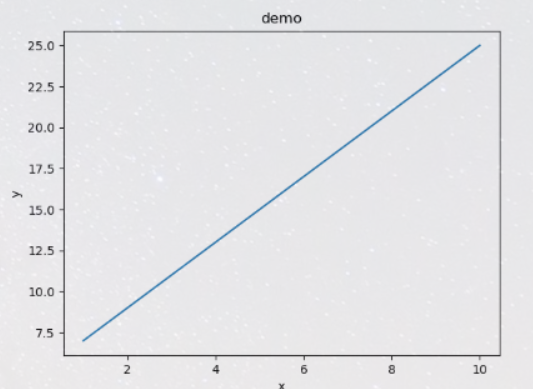np.arange() 函数创建 x 轴上的值。y 轴上的对应值存储在另一个数组对象 y 中。 这些值使用 matplotlib 软件包的 pyplot 子模块的 plot() 函数绘制。

`'-'`实线样式
`'--'`短横线样式
`'-.'`点划线样式
`':'`虚线样式
`'.'`点标记
`','`像素标记
`'o'`圆标记
`'v'`倒三角标记
`'^'`正三角标记
`'<'`左三角标记
`'>'`右三角标记
`'1'`下箭头标记
`'2'`上箭头标记
`'3'`左箭头标记
`'4'`右箭头标记
`'s'`正方形标记
`'p'`五边形标记
`'*'`星形标记
`'h'`六边形标记 1
`'H'`六边形标记 2
`'+'`加号标记
`'x'`X 标记
`'D'`菱形标记
`'d'`窄菱形标记
`'|'`竖直线标记
`'_'`水平线标记

`'b'`蓝色
`'g'`绿色
`'r'`红色
`'c'`青色
`'m'`品红色
`'y'`黄色
`'k'`黑色
`'w'`白色
``````import numpy as np
from matplotlib import pyplot as plt

x = np.arange(1,11)
y =  2  * x +  5
plt.title("Matplotlib demo")
plt.xlabel("x axis caption")
plt.ylabel("y axis caption")
plt.plot(x,y,"ob")
plt.show()
``````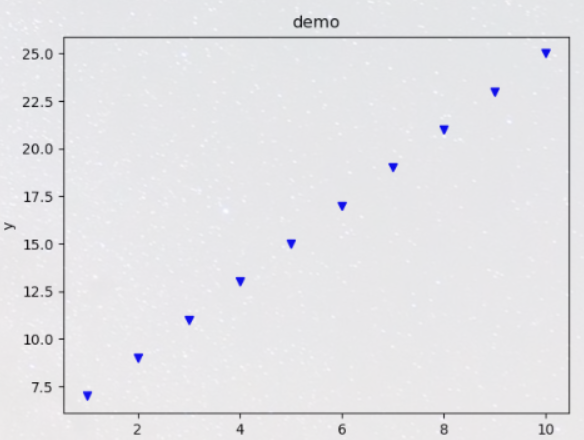### 绘制正弦波

``````# 绘制正弦波
x = np.arange(0, 3 * np.pi, 0.1)
y = np.sin(x)
plt.title("sin(x)")
plt.plot(x, y, "--r")
plt.show()
``````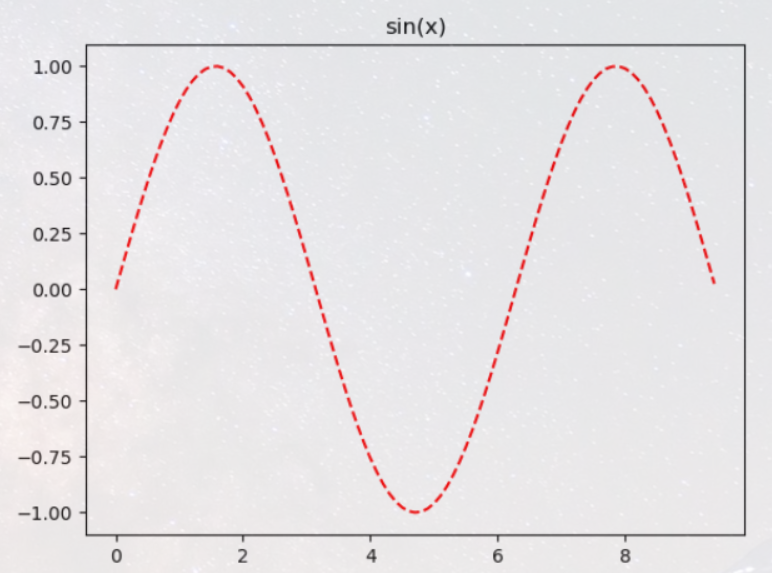### subplot()

subplot() 函数允许你在同一图中绘制不同的东西。

``````# subplot()同一图中绘制不同的东西 ,
x = np.arange(0, 3 * np.pi, 0.1)
y_sin = np.sin(x)
y_cos = np.cos(x)
# 建立 subplot 网格，高为 2，宽为 1
# 激活第一个 subplot
plt.subplot(2, 1, 1)
# 绘制第一个图像
plt.plot(x, y_sin)
plt.title("sin(x)")
# 将第二个 subplot 激活，并绘制第二个图像
plt.subplot(2, 1, 2)
plt.plot(x, y_cos)
plt.title(np.cos(x))
# 展示图象
plt.show()
``````

### bar()

pyplot 子模块提供 bar() 函数来生成条形图。

``````x = [5, 8, 10]
y = [12, 16, 6]
x2 = [6, 9, 11]
y2 = [6, 15, 7]
plt.bar(x, y, align='center')
plt.bar(x2, y2, color='g', align='center')
plt.title('Bar graph')
plt.ylabel('Y axis')
plt.xlabel('X axis')
plt.show()
``````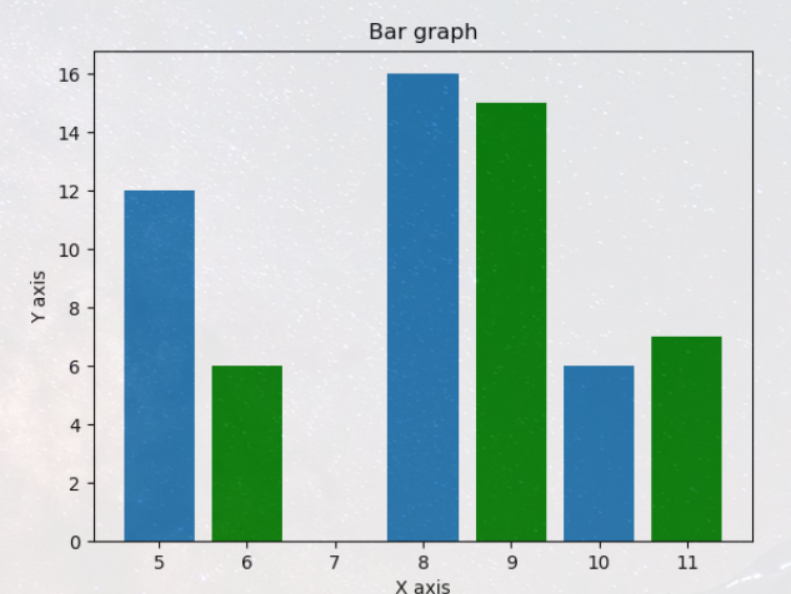### numpy.histogram()

numpy.histogram() 函数是数据的频率分布的图形表示。 水平尺寸相等的矩形对应于类间隔，称为 bin，变量 height 对应于频率。

numpy.histogram()函数将输入数组和 bin 作为两个参数。 bin 数组中的连续元素用作每个 bin 的边界。

``````a = np.array([22, 87, 5, 43, 56, 73, 55, 54, 11, 20, 51, 5, 79, 31, 27])
np.histogram(a, bins=[0, 20, 40, 60, 80, 100])
hist, bins = np.histogram(a, bins=[0, 20, 40, 60, 80, 100])
print(hist)
print(bins)
``````
``````[3 4 5 2 1]
[  0  20  40  60  80 100]
``````

### plt()

Matplotlib 可以将直方图的数字表示转换为图形。 pyplot 子模块的 plt() 函数将包含数据和 bin 数组的数组作为参数，并转换为直方图。

``````a = np.array([22, 87, 5, 43, 56, 73, 55, 54, 11, 20, 51, 5, 79, 31, 27])
plt.hist(a, bins=[0, 20, 40, 60, 80, 100])
plt.title("histogram")
plt.show()
``````